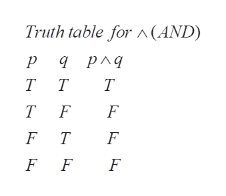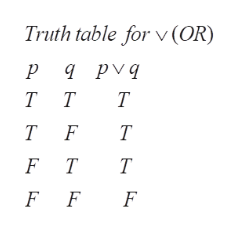Hello, I'd like to know how to make a truth table for the following equation, thanks! (P ⋀ Q) ⋁ (P ⋀ R)

Question

Hello, I'd like to know how to make a truth table for the following equation, thanks!

(P ⋀ Q) ⋁ (P ⋀ R)

Step 1

To construct the required truth table

Step 2

Basic truth tables: for ANDhelp_outlineImage TranscriptioncloseTruth table for A(AND) ра рлд тт Т T F F F T F F F fullscreen
Step 3

Basic truth table f...help_outlineImage TranscriptioncloseTruth table for v(OR) ра pуд рvд Т T T F T F T Т F F F fullscreen

Want to see the full answer?

See Solution

Want to see this answer and more?

Our solutions are written by experts, many with advanced degrees, and available 24/7

See Solution
Tagged in

Math